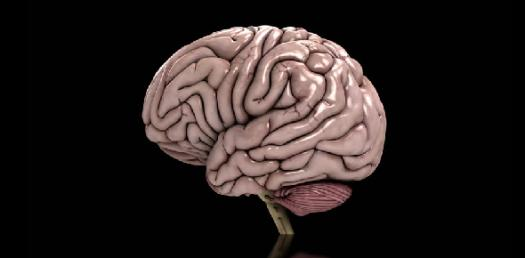5 QuestionsThis quiz make your brain squeeze! PLAY IT OR JUST LEAVE IT!

Related Topics
• 1.
• A.

Janeeeeeeeeeeeee

• B.

Janeeeeeeee Emilyyyyyyyyy

• C.

Jane Emily

• 2.
2+2=.....
• A.

#F

• B.

4

• C.

Fish

• 3.
1+1=...
• A.

Window

• B.

1

• C.

2

• 4.
Who is Kevin?
• A.

My friend

• B.

God creatures

• C.

People

• 5.
....=03+02....=20+02....=05+04....=10+02___+
• A.

05+22+09+12=Evil

• B.

05+22+09+12=48

• C.

E+V+I+L=EVIL